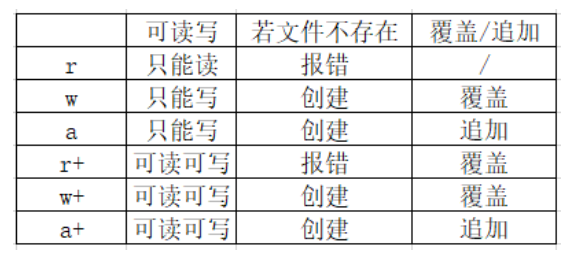python中读写txt文件，首先得打开文件，即使用open()函数，

``````lastpath1 = r'D:apache-jmeter-4.0insrcWaveId.txt'
file1 = open(lastpath,'r'')
````````````path1=r'D:Document	est.txt'
file1=open(path1,'r')
print(content1)
file1.close()  #文件打开，使用完毕后需要关闭
``````

``````path2=r'D:Document	est.txt'
file2=open(path1,'r')
print(content2)
file2.close() #文件打开，使用完毕后需要关闭
``````

``````path3=r'D:Document	est.txt'
file3=open(path3,'r')
print(content3)
file3.close() #文件打开，使用完毕后需要关闭
``````

``````['one
', 'two
', 'three
', 'four
', 'five']
``````

``````f = open("test.txt", "r")
line = line.strip('
')  #去掉列表中每一个元素的换行符
print(line)
f.close()
``````

1、文件中写入内容，首先需要打开文件
2.wirte()写入后默认不换行

``````path2 = r'D:Document	est2.txt'
file2 = open(path2,'w+')
file2.write('aaa')
b = 'ccc'
file2.write(b)
``````